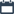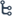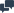Welcome to Instructbd

## Monday, 6 June 2016

•01:52:00
•••1 comment

## [Book] Fourier and Laplace Transforms By R.j. Beerends, H.G. ter Morsche, J.C. Van den Berg and E.M. Van de Vrie

#### Fourier and Laplace transforms are examples of mathematical operations which can play an important role in the analysis of mathematical models for problems originating from a broad spectrum of fields. These transforms are certainly not new, but the strong development of digital computers has given a new impulse to both the applications and the theory. The material in this book is subdivided into parts. Each part consists of a number of coherent chapters covering a specific part of the field of Fourier and Laplace transforms. Sections contain such items as definitions, theorems, examples, and so on. These are clearly marked in the left margin, often with a number attached to them. For almost all theorems proofs are given following the heading Proof. The end of a proof is indicated by a right-aligned black square: . In some cases it may be wise to skip the proof of a theorem in a first reading, in order not to lose the main line of argument. The proof can be studied later on. Examples are sometimes included in the running text, but often they are presented separately. In the latter case they are again clearly marked in the left margin (with possibly a number, if this is needed as a reference later on). The end of an example is indicated by a right-aligned black triangle: . Mathematical formulas that are displayed on a separate line may or may not be numbered. Only formulas referred to later on in the text have a number (right-aligned and in brackets).

Title: Fourier and Laplace Transforms
Author : R.j. Beerends, H.G. ter Morsche, J.C. Van den Berg and E.M. Van de Vrie
Published: 2003

Total Pages: 459

Size : 02 MB

#### Content:

• Applications and Foundations
• Fourier Series
• Laplace Transformers
• Discrete Transforms
• Literature
• Tables of Transformers and Properties
• Index

(you can also read this book online)

•01:33:00
•••1 comment

## [Book] A First Course in Differential Equations with Modeling Applications By Dennis G. Zill

#### This book is mainly focus on Differential Equations and Modeling application. The authors of this book tried to write this book easily for better understand of the students. But Beginner level students have been facing some problems to solve this math because most of the section are formed with Partial Differential, First order Differential Equations, Second Order or Higher Order Differential Equations and Boundary Equations problems. So that If you have don't have any previous idea about differential method you are not able to understand the math of this book and if you don't able to solve these differential problem from these book , you can't learn the modeling application which are solved using differential method. Moreover,  In this book, Authors do not leave much space for explanations and examples, the explanations this book does provide are extremely difficult to follow, and the examples given are mostly skipping over a majority of the steps involved in solving the problems. For this reason, Beginners level students needs a guide or decent teacher to helps you solve this math. On top of everything, You must have interest in learning. If you want to learn something than you have to practicing the math more and more time. More practicing makes a student perfect.

Title: A First Course in Differential Equations with Modeling Applications
Author : Dennis G. Zill

Last Published: 2013

Edition: 10th

Total Pages: 489

Size : 12 MB

#### Content:

• Introduction To Differential Equations
• First-Order Differential Equations
• Modeling With First-Order Differential Equations
• High-Order Differential Equations
• Modeling with Higher-Order Differential Equations
• Series Solutions of Linear Equations
• The Laplace Transform
•  System of Linear First-Order Differential Equations
• Numerical Solutions of Ordinary Differential Equations
• Appendixes

(you can also read this book online)